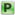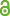Hauptmenü
• Autor
• Berkes, István
• TitelStrong approximation and a central limit theorem for St. Petersburg sums
• Datei
• LicenceCC BY
• Zugriffsrechte• AbstractThe St. Petersburg paradox (Bernoulli 1738) concerns the fair entry fee in a game where the winnings are distributed as  $$P(X = 2^k) = 2^{−k} , k = 1, 2, . . .$$. The tails of X are not regularly varying and the sequence $$S_n$$ of accumulated gains has a remarkable asymptotic behavior: as Martin-Löf (1985) and Csörgő and Dodunekova (1991) showed, $$S_n /n − log_2 n$$ has a class of semistable laws as subsequential limit distributions. This has led to a clarification of the paradox and an interesting and unusual asymptotic theory in past decades. In this paper we prove that $$S_n$$ can be approximated by a semistable Lévy process $$\{L(n), n ≥ 1\}$$ with a.s. error $$O(\sqrt{n}(log n)^{1+ε})$$ and, surprisingly, the error term is asymptotically normal, exhibiting an unexpected central limit theorem in St. Petersburg theory.